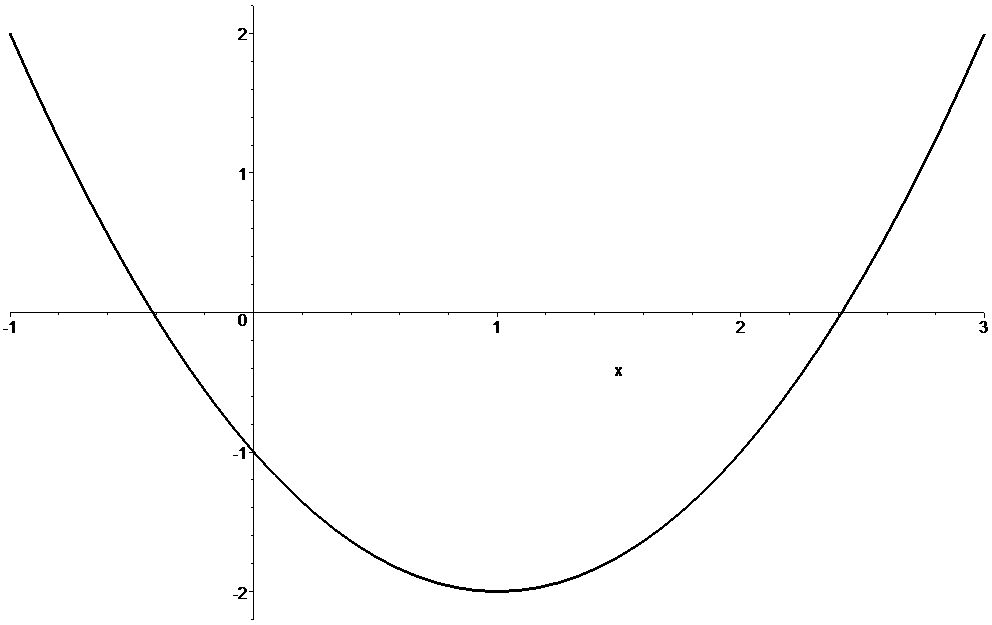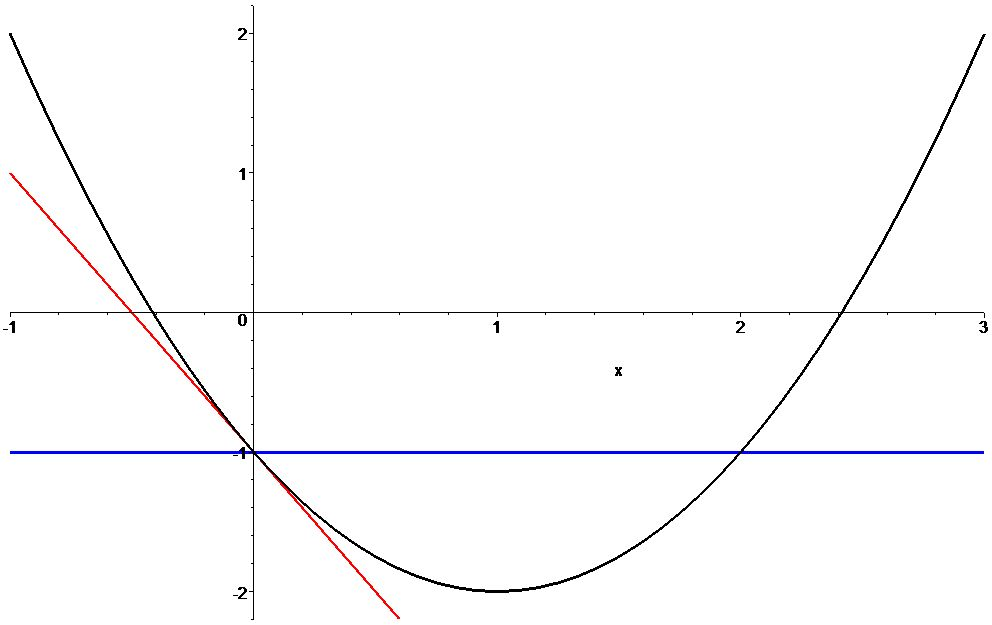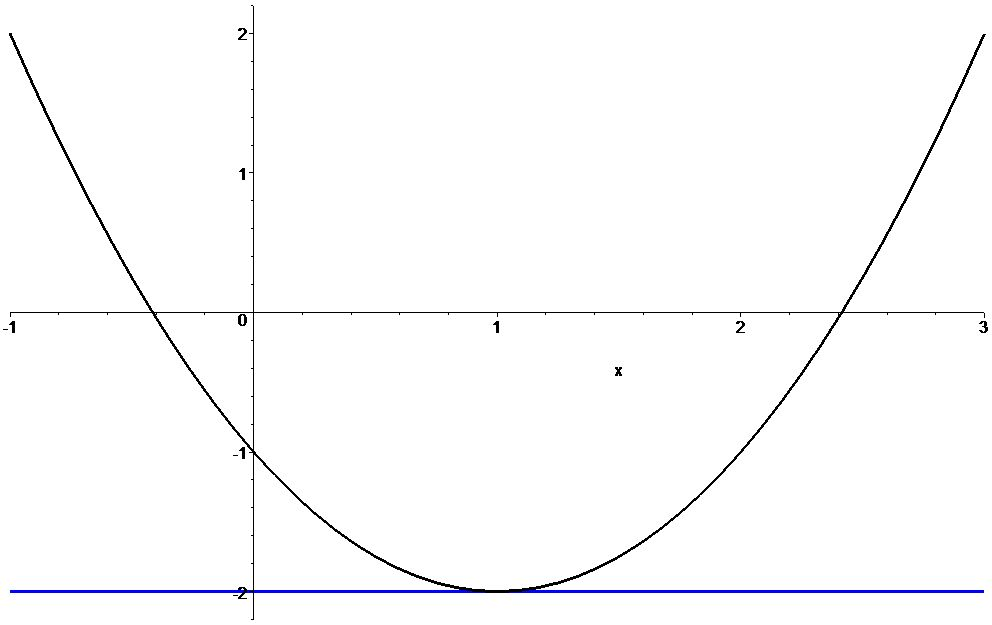## Section7.4Discussion of Approximations using Power Series

Why should you care about power series? One reason is because they allow us to approximate functions at a point to any desired accuracy.

For instance, consider the function $$h(x)=-1-2x+x^2\text{.}$$ Draw its graph.Figure 7.4.1. The graph of the polynomial $$h(x)=-1-2x+x^2\text{.}$$

Notice that we have chosen a particularly simple function for this example, a polynomial. Just by looking at it; you can see that the function already has the form of a power series with only three nonzero terms. Its power series, expanded around the origin, is just the algebraic expression for the function itself. Obviously, there is no reason for you to approximate a function this simple. We are using this simple example so that you can think about the separate terms quickly and easily in your head. In Section 7.2 and Section 7.3 you have the opportunity to work with the power series for $$\sin\theta\text{,}$$ which has an infinite number of terms. You will have many chances to see why power series approximations are useful for more complicated functions later in this text.

At the origin $$x=0\text{,}$$ the value of the function is $$-1\text{.}$$ So, the zeroth order approximation is just the constant function $$-1\text{.}$$ It agrees with the value of the function exactly at $$x=0\text{,}$$ but otherwise is a pretty lousy approximation, see Figure 7.4.2. The slope of this graph at $$x=0$$ is given by the derivative, namely $$2\text{.}$$ The linear approximation at the origin to this function is therefore the straight line $$y=-1-2x\text{.}$$ Where is this linear approximation for this function a “good” approximation?Figure 7.4.2. The graph of the polynomial $$h(x)=-1-2x+x^2$$ (black) together with its zeroth order (constant) approximation at the origin (blue) and its first order (linear) approximation at the origin (red).

The approximation that we found above, which was a first order series expansion around $$x=0$$ isn't a very good approximation near $$x=1\text{.}$$ We can find a better approximation if we choose to expand around another point, say $$x=1\text{,}$$ see Figure 7.4.3. The expansion of $$h$$ around $$1$$ has just two terms, namely $$h(x)=-2+(x-1)^2\text{.}$$ The absence of a linear term in this expansion tells us that the graph is horizontal at $$x=1\text{.}$$Figure 7.4.3. The graph of the polynomial $$h(x)=-1-2x+x^2$$ (black) together with its zeroth order (and linear) approximation at $$x=1$$ (blue).

You can explore power series approximations for yourself using the applet in Section 7.5